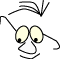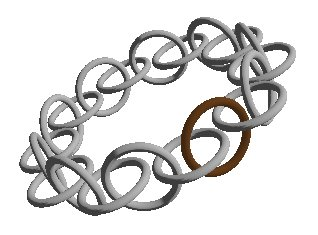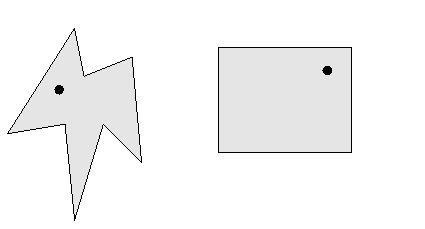TexturOpenGL>Selection

# Selection by pointing

WhatSelection of an object in a scene by pointing at it

The question we raise is how should we identify a part of a scene by pointing at it. We can easily see that this problem is different in 2D and 3D. This module attempts to investigate this in 3 situations:

1. general algorithm to check if a point is inside a polygon
2. how to hit something in a transformed 2D environment
3. how to hit something in a 3D scene in OpenGL

The illustration to the right shows a identified and hilited torus in a chain of toruses.

## General algorithm

In general we can solve the problem by identifying a polygon that contains our click-point. We do assume that polygons does not overlap. The polygons we test our point against should be convex and concave.Testing a point, p, against a rectangle, r, is trivial.

``` inside =(p.x < r.right)&&(p.x > r.left)&&(p.y < p.bottom)&&(p.y > p.top);
```

The following two methods are a sketch for a general inside test. The first, Intersection, finds possible intersections between lines. Written i c.

_intersection.c

The other method, Inside, may be used to test if a point is inside a polygon:

_inside.c
```BOOL Inside(CPoint p)
{
/* Have we hit the polygon in pointarray POL ?
assume POL closed, POL == POL [POL.GetSize()-1]
if we have a sorrounding box for the polygon
we should test this first
For instance like this:
if(!m_BigR.PtInRect(p))
return FALSE;
*/
// Count intersections to the left of p
// odd is hit, even is miss
int pix;
CPoint p1,p2,p3,p4,ps;
BOOL Inside=FALSE;
p1.x=-<a number smaller than smallest x in POL >;
p1.y=p.y;
p2.x=<a number greater than greatest x in POL >;
p2.y=p.y;
for(pix=0;pix< POL.GetSize()-1;pix++)
{
p3= POL [pix];
p4= POL [pix+1];
if(Intersection(p1,p2,p3,p4,ps))
if (ps.x < p.x )
Inside=!Inside;
}
return Inside;
}
```

## Transformed 2D

The situation is getting a little more tricky if we draw in 2D and use transformations (rotate, translate, scale) when we draw. Most drawing environments, like Java SDK and .net (C++,VB,C#). In Java we find the transformation routines in class graphics2D.

We can use the strategy described in 2D transformations, and build a matrix for drawing. When we click on a drawing that has been transformed, we must transform the click point from screen coordinates to "model-coordinates" by the inverse transformation matrix and then do the test.

## 3D

In 3D the reasoning gets more complicated.

• The scene is transformed to a coordinate system that involves 3 D. We can still get the inverse transformation matrix, but the setting is more complex since it also involves a perspective transformation.
• We will have problems deciding which component in the scene is closest to the eye. We cannot in general anticipate any relations between components regarding distance from eye.

In OpenGL this situation is resolved by a strategy that is involved in the rendering process:

1. The user points at the scene, clicks
2. The program generates a small clipping volume with centre in the mouse click
3. The program repeats the rendering of the scene that involves the new small clipping volume. Nothing is changed on the screen during this "extra" rendering.
4. During this rendering we can give names to parts of the scene that are potential hit targets. The names that are active during clipping are stored by OpenGL during the rendering.
5. After the extra rendering we can pick up all the names that has been noted, and we can read their z-coordinates (distance as seen from the eye)

An implementation of this strategy could be like this:

```private int pickSelected(GL gl,int x,int y)
{
GLU glu = new GLU();
int BUFSIZE=1024;
int hits;
int viewport[]=new int;// to store current viewport
int closestix;
int ix;
IntBuffer buf=BufferUtil.newIntBuffer(BUFSIZE);
gl.glGetIntegerv(GL.GL_VIEWPORT,viewport,0);// get viewport
gl.glSelectBuffer(BUFSIZE,buf);// tell OGL where to report
gl.glRenderMode(GL.GL_SELECT);// as opposed to GL_RENDER
gl.glInitNames();
gl.glPushName(-1);
gl.glMatrixMode(GL.GL_PROJECTION);
gl.glPushMatrix();
// set up a small clipping pyramid
glu.gluPickMatrix(x,(viewport-y),5.0f,5.0f,viewport,0);
// same view as we see it, same as in reshape
glu.gluPerspective(45.0f,(float)viewport / (float)viewport,
1.0f, 200.0f);
gl.glMatrixMode(GL.GL_MODELVIEW);
//--------------------
// draw it without rendering
drawToruses(gl,GL.GL_SELECT);
//----------------
gl.glMatrixMode(GL.GL_PROJECTION);
gl.glPopMatrix();
gl.glMatrixMode(GL.GL_MODELVIEW);
hits=gl.glRenderMode(GL.GL_RENDER);// gives me a hitcount
// process the ix+1 hits
// selectBuf contents:
//  ix  : number of names on the hitstack when hit occurred
//  ix+1: min z-depth of "object" associated with name, relative
//  ix+2: max z-depth of "object" associated with name, relative
//  ix+3: the name, ie index, assosiated with the hit
//  etc
if(hits ==0)
return -1;
else
{
// we have hit one or more "objects"
// if only one we simply select it
if(hits ==1)
return buf.get(3);//the only one in store
// more than one is hit.  Which should we select ?
// The closest one,
// go for the one which closest part is closest
closestix=0;
for(ix=1;ix<hits;ix++)
if(buf.get(4*ix+1) < buf.get(4*closestix+1))
closestix=ix;
return buf.get(4*closestix+3);
}
}```

The display routine uses this method according to this scheme:

```public void display(GLAutoDrawable drawable) {
GL gl = drawable.getGL();
// Enable VSync
gl.setSwapInterval(1);
// Clear the drawing area
gl.glClear(GL.GL_COLOR_BUFFER_BIT | GL.GL_DEPTH_BUFFER_BIT);

if(m_selecting)// we have pressed right mousebutton
m_hilited=pickSelected(gl,(int)m_lastMouseX,(int)m_lastMouseY);